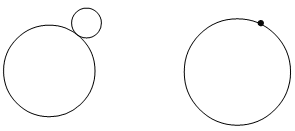# 4.11 对小而快的对象使用Ray-Traced进行碰撞检测

## 工作原理

myModel = XNAUtils.LoadModelWithBoundingSphere(ref modelTransforms, "tank", Content);

private bool RayCollision(Model model, Matrix world, Vector3 lastPosition, Vector3 currentPosition)
{
BoundingSphere modelSpere = (BoundingSphere)model.Tag;
BoundingSphere transSphere = TransformBoundingSphere(modelSpere, world);
} 

Vector3 direction = currentPosition - lastPosition;
float distanceCovered = direction.Length();
direction.Normalize(); 

Ray ray = new Ray(lastPosition, direction);

bool collision = false;
float? intersection = ray.Intersects(transSphere);
if (intersection != null)
if (intersection <= distanceCovered)
collision = true;
return collision; 

Intersection方法有点特别。如果发生碰撞，这个方法返回碰撞点和用来定义Ray的点之间的距离。但是，如果Ray和包围球没有发生碰撞，这个方法返回null。所以你需要一个 float?变量而不是float，因为它需要能够存储null值。

### 大而快的物体## 代码

protected override void LoadContent()
{
device = graphics.GraphicsDevice;
basicEffect = new BasicEffect(device, null);
cCross = new CoordCross(device);
myModel = XNAUtils.LoadModelWithBoundingSphere(ref modelTransforms, "tank", Content);
} 

private bool RayCollision(Model model, Matrix world, Vector3 lastPosition, Vector3 currentPosition)
{
BoundingSphere modelSpere = (BoundingSphere)model.Tag;
BoundingSphere transSphere = XNAUtils.TransformBoundingSphere(modelSpere, world);

Vector3 direction = currentPosition - lastPosition;
float distanceCovered = direction.Length();
direction.Normalize();

Ray ray = new Ray(lastPosition, direction);

bool collision = false;
float? intersection = ray.Intersects(transSphere);
if (intersection != null)
if (intersection <= distanceCovered)
collision = true;
return collision;
} 

2006 - 2023，推荐分辨率1024*768以上，推荐浏览器Chrome、Edge等现代浏览器，截止2021年12月5日的访问次数：1872万9823 站长邮箱